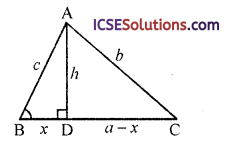Students appreciate clear and concise ICSE Class 9 Maths Solutions S Chand Chapter 10 Pythagoras Theorem Ex 10(B) that guide them through exercises.

## S Chand Class 9 ICSE Maths Solutions Chapter 10 Pythagoras Theorem Ex 10(B)

Question 1.
ABCD is a square, prove that AC² = 2AB².
Solution:
Given : ABCD is a square in which AC is its diagonalTo prove : AC² = 2Ab²
Proof: In ∆ABC, ∠B = 90°
∴ AC² = AB² + BC² (Pythagoras Theorem)
= (AB)² + (AB)²
{∵ AB = BC sides of the square}
= 2Ab²
Hence AC² = 2AB²

Question 2.
In the figure, AB = BC = CA = 2a and segment AD ⊥ side BC. Draw that
(i) AD = a$$\sqrt{3}$$
(ii) area of ∆ABC = a²$$\sqrt{3}$$Solution:
Given : In ∆ABC,
AB = BC = CA = 2aTo prove :
(i) AD = a$$\sqrt{3}$$
(ii) area of ∆ABC = a²$$\sqrt{3}$$
Proof:
(i) ∵ The sides of ∆ABC are equal (each = 2d)
∴ It is an equilateral triangle
∴ AD bisects BC at D
i.e. BD = DC = a
Now in right ∆ABD
AB² = AD² + BD² (Pythagoras Theorem)
⇒ (2a)² = AD² + (a)²
⇒ 4a² = AD² + a² ⇒ AD² = 4a² – a²
⇒ AD² = 3a² ⇒ AD = $$\sqrt{3}$$

(ii) Now area of ∆ABC = $$\frac { 1 }{ 2 }$$ base x altitude
= $$\frac { 1 }{ 2 }$$ BC x AD
= $$\frac { 1 }{ 2 }$$ (2a) ($$\sqrt{3}$$a)
= $$\sqrt{3}$$ a² = a²$$\sqrt{3}$$
Hence proved.

Question 3.
In the figure, prove that Ab² – AD² = CD² – CB².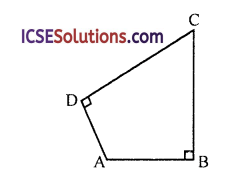Solution:
∠B = 90° and ∠D = 90°
To prove : Ab² – AD² = CD² – CB²
Construction : Join ACProof: In right ∆ABC, ∠B = 90°
∴ AC² = AB² + BC² … (i)
(Pythagoras Theorem) Similarly in right ∆ADC,
AC² = AD² + CD² … (ii)
From (i) and (ii),
AB² + BC² = AD² + CD²
⇒ AB² – AD² = CD² – BC²
⇒ AB² – AD² = CD² – CB²
Hence proved.Question 4.
In a ∆ABC, AD ⊥ BC. Prove that AB² + CD² = AC² + BD².
Solution:
Given : In ∆ABC, AD ⊥ BCTo prove : AB² + CD² = AC² + BD²
Proof: In right ∆ABD (∵ AD ⊥ BC)
AB² = BD² + AD² (Pythagoras Theorem)
⇒ AD² = AB² – BD² … (i)
Similarly in right ∆ACD
⇒ AD² = AC² – CD² … (ii)
From (i) and (ii),
AB² – BD² = AC² – CD²
∴ AB² + CD² = AC² + BD²
Hence proved.

Question 5.
In a quadrilateral ABCD, the diagonals AC, BD intersect at right angles. Prove that Ab² + CD² = BC² + DA²
[No marks will be given if it is assumed that ABCD is either a rhombus or a square].
Solution:
Given: In quadrilateral ABCD, diagonals AC and BD intersect each other at right angles at O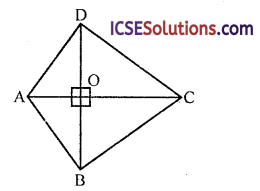To prove : AB² + CD² = BC² + DA²
Proof: In right ∆ABO,
AB² = AO² + BO² (Pythagoras Theorem)
Similarly in right ∆BOC,
BC² = BO² + CO²
In right ∆COD
CD² = CO² + DO²
and in right ∆AOD
DA² = AO² + DO²
Now AB² + CD² = AD² + BO² + CO² + DO² … (i)
and BC² + DA² = BO² + CO² + AO² + DO²
= AO² + BO² + CO² + DO² … (ii)
From (i) and (ii),
AB² + CD² = BC² + DA²
Hence proved.

Question 6.
In ∆ABC, ∠B = 90° and D is mid-point of BC. Prove that
(i) AC² = AD² + 3CD²
(ii) BC² = 4 (AD² – AB²)
Solution:
Given : In ∆ABC, ∠B = 90°
D is mid-point of BCTo prove :
(i) AC² = AD² + 3CD²
(ii) BC² = 4 (AD² – AB²)
Proof:
In right ∆ABD,
AD² = AB² + BD² (Pythagoras Theorem)
⇒ AD² = AB² + ($$\frac { 1 }{ 2 }$$BC)² (∵ D is mid-point of BC)
⇒ AD² = AB² + $$\frac{B C^2}{4}$$
⇒ 4AD² = 4AB² + BC²
(i) and in right ∆ABC
(ii) AC² = AB² + BC²
= AB² + (2CD)² (∵D is mid-point of BC)
= AB² + 4CD²
= (AD² – BD²) + 4CD² {∵ AD² = AB² + BD²}
= AD² – CD² + 4CD² (∵ BD = CD)
Hence proved.

Question 7.
The side BC of a square ABCD is produced to any point E. Prove that AE² = 2BC. BE + CE².
Solution:
Given : ABCD is a square whose side BC is produced to E
EA is joined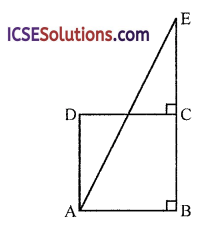To prove : AE² = 2BC.BE + CE²
Proof: In right ∆ABE
AE² = AB² + BE² = AB² + (BC + CE)²
= AB² + BC² + CE² + 2BC.CE
= BC² + BC² + CE² + 2BC (BE – BC)
= 2BC² + CE² + 2BC.BE – 2BC²
= 2BC.BE + CE²
Hence proved.Question 8.
ABCD is a rhombus. Prove that AC² + BD² = 4AB².
Solution:
Given : In rhombus ABCD, diagonals AC and BD bisect each other at right angle at O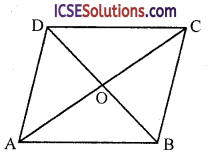In right ∆AOB, ∠AOB = 90°
∴ AB² = AO² + OB²
⇒ AB² = ($$\frac { 1 }{ 2 }$$AC)² + ($$\frac { 1 }{ 2 }$$²BD
⇒ AB² = $$\frac { 1 }{ 4 }$$ AC² + $$\frac { 1 }{ 4 }$$BD²
⇒ 4AB² = AC² + BD²
Hence AC² + BD² = 4AB²
Hence proved.

Question 9.
In the figure, ∠B of ∆ABC is an acute angle and AD ⊥ BC. Prove that
AC² = AB² + BC² – 2BC.BDSolution:
Given : In ∆ABC, ∠B is an acute angle AD ⊥ BC
To prove : AC² = AB² + BC² – 2BC.BD
Proof: In right ∆ABD
AB² = AD² + BD² (Pythagoras Theorem)
⇒ AD² = AB² – BD² … (i)
= AB² – BD² + (BC – BD)²
= AB² – BD² + BC² + BD² – 2BC.BD
= AB² + BC² – 2BC.BD
Hence proved.

Question 10.
In a quadrilateral ∆BCD, ∠B = 90° and AD² = AB² + BC² + CD². Prove that ∠ACD = 90°.
Solution:
Given : In quadrilateral ABCD, ∠B = 90° and AD² = AB² + BC² + CD²To prove : ∠ACD = 90°
Proof: In right ∆ABC
AC² = AB² + Bc² … (i)
(Pythagoras Theorem)
∵ AD² = AB² + BC² + CD² (given)
AD² = AC² + CD² [From (i)]
∴ In ∆ACD,
∠ACD = 90°
(Converse of Pythagoras Theorem)
Hence proved.Question 11.
ABC is a triangle right angled at A and p is the length of the perpendicular from A on BC. Show that
(i) pa = bc Hence deduce that
(ii) $$\frac{1}{p^2}=\frac{1}{b^2}+\frac{1}{c^2}$$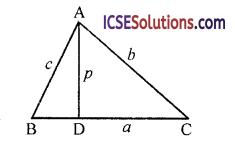Solution:
Given : In ∆ABC, ∠A = 90°
AD = p, AB = c, BC = a and AC = bSolution:
(i) pa = bc
(ii) $$\frac{1}{p^2}=\frac{1}{b^2}+\frac{1}{c^2}$$
Proof: In right ∆ABC, ∠A = 90°
∴ BC² = AB² + AC² (Pythagoras Theorem)
a² = c² + b² = b² + c² …. (i)

(i) Area of ∆ABC = $$\frac { 1 }{ 2 }$$ base x altitude
= $$\frac { 1 }{ 2 }$$ BC x AD
= $$\frac { 1 }{ 2 }$$ ap
and also area of ∆ABC = $$\frac { 1 }{ 2 }$$ AB x AC
= $$\frac { 1 }{ 2 }$$ c.b
∴ $$\frac { 1 }{ 2 }$$ pa = $$\frac { 1 }{ 2 }$$ bc ⇒ Pa = bc

(ii) ∵ pa = bc
Squaring both sides,
p²a² = b²c²
p² (b² + c²) = b²c² [from (i)]
p² = $$\frac{b^2 c^2}{b^2+c^2} \Rightarrow \frac{1}{p^2}=\frac{b^2+c^2}{b^2 c^2}$$
⇒ $$\frac{1}{p^2}=\frac{b^2}{b^2 c^2}+\frac{c^2}{b^2 c^2}$$
⇒ $$\frac{1}{p^2}=\frac{1}{c^2}+\frac{1}{b^2}$$
Hence proved.

Question 12.
In the given figure, ∠B is acute and segment
AD ⊥ side BC. Show that
(i) b² = h² + a² + x² – 2ax
(ii) b² = a² + c² – 2ax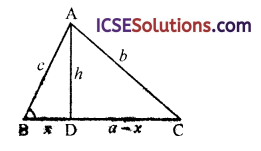Solution:
Given : In ∆ABC, ∠B acute angle